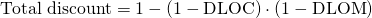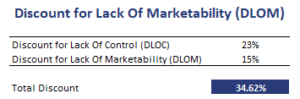## Discount for Lack of Marketability (DLOM)

The Discount for Lack of Marketability (DLOM) studies or captures the fact that a stake in a company cannot always be sold easily. Often, when a DLOM is applied, a Discount for Lack of Control (DLOC) is also applied. Fortunately, we can easily calculate the total discount that should be applied using a single formula.

On this page, we discuss the marketability discount for private companies and the marketability discount calculation methods available. We also include an example of how to calculate the total discount once we have both the DLOC and the DLOM.

## DLOM determinants

The DLOM tends to decrease when an IPO or firm sale is pending, a payment of dividends is planned, or when there is a greater pool of potential buyers. The DLOM will be higher when there are contractual restrictions on selling the shares, the company is riskier and its value is more uncertain. The DLOM is almost always applied when valuing private companies.

## Discount for Lack of Marketability calculation

The discount for lack of marketability calculation can be based on three different approaches.

• The first approach uses the price of restricted shares. In that case, the price of the restricted shares is compared to the price of the publicly traded shares.
• The second approach estimates the DLOM using the price of a put option divided by the stock price, where the put option used is ATM (at the money). The advantage of this second approach is that the estimated risk of the company can be factored in (via the volatility). The disadvantage is that a put option provides a certain selling price, but it ignores the actual liquidity.
• The third approach compares the price of pre-IPO shares to the price of the post-IPO shares. The drawback of this approach is that the post-IPO shares may also be higher for other reasons (e.g. lower risk due to higher disclosure).

In the example below, we combine the discount for lack of control and marketability to calculate the total discount. It is important to keep in mind that both measures are applied in a sequential process. As a consequence, both discounts are multiplicative.## DLOC and DLOM example

In the following figure, we illustrate how the DLOC and DLOM are combined to calculate the total discount. It is important to note that we are not adding the DLOC and DLOM. Instead, we apply them in a sequential manner.## Summary

We discussed the DLOM, a method to adjust the valuation of a private company for the fact that selling the interest will not be easy.

### DLOM calculator

Want to have an implementation in Excel? Download the Excel file: DLOM